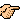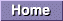# MH-plus project \$B\$N\$Z!<\$8(B

### MH \$B\$N(B \$BF|K\8l(B READ-ME (\$B;CDjHG(B) \$B!A(B by MH-plus project

MH \$B\$r=i\$a\$F;H\$*\$&\$H\$9\$kJ}\$X\$N!"(BMH \$B<+BN\$K\$D\$\$\$F\$N4JC1\$J@bL@\$G\$9!#(B MH-JP \$B\$N%\$%s%9%H!<%k\$K4X\$7\$F\$O!"(BINSTALL.JP \$B\$r;2>H\$7\$F2<\$5\$\$!#(B

\$B>0!"(BMH \$B\$K4X\$7\$F\$G\$9\$,!"(B

• distribution \$BFb\$N(B
• papers/multifarious/appendixA.tex
• papers/multifarious/text.tex
• papers/beginners/beginners.tex
\$BB>!#(B
• comp.mail.mh \$B\$KEj9F\$5\$l\$k!"(BBill Wohler \$B;a(B(wohler@newt.com)\$B\$K\$h\$k(B FAQ \$B!V(BSubject: MH Frequently Asked Questions (FAQ) with Answers\$B!W(B
• http://www.ics.uci.edu/%7Emh/ \$B\$d(B MH book (\$BDL>N(B:\$B%?%3K\(B)\$B\$N%*%s%i%\$%sHG(B(Html\$BHG(B) ( http://www.ics.uci.edu/%7Emh/book/\$B\$J\$I\$G8x3+\$5\$l\$F\$\$\$^\$9(B)
\$B\$J\$I\$,;29M\$K\$J\$j\$^\$9!#FC\$K!"(BMH \$B\$r%\$%s%9%H!<%k\$7\$h\$&\$H\$9\$k:]\$K\$O!"(B \$BM=\$a(B FAQ \$B\$K\$OL\\$rDL\$7\$F\$*\$/\$3\$H\$r\$*4+\$a\$7\$^\$9!#(B

#### MH \$B\$H\$O(B?

mailer \$B%f!<%6%\$%s%?!<%U%'!<%9\$G!"(B"Mail Handler"\$B\$NN,\$G\$9(B(\$B85!9(B Rand \$B

#### MH \$B\$NNr;K(B

\$B85!9!"(BMH \$B\$O(B Rand \$BpJs2J3X2J(B(UCI)\$B\$,!"(BCSnet(Computer Science Network)\$B2CF~D>8e\$K\$=\$N%3%T!<\$rF~

#### MH \$B\$N4pK\9=@.35G0(B

MH \$B\$O!V%a!<%k4XO"\$N5!G=\$rA4\$FM-\$7\$?0l\$D\$N5pBg\$J%W%m%0%i%`!W\$G\$O\$"\$j\$^\$;\$s!#(B \$B0l\$D0l\$D\$,\$"\$kL\E*\$r9T\$J\$&!">.\$5\$J%3%^%s%I%W%m%0%i%`\$N=89gBN\$G\$9!#(B \$B\$=\$N\$?\$a\$+!">/\$7\$H\$C\$D\$-Fq\$\$\$+\$bCN\$l\$^\$;\$s\$,!"(BUNIX \$B\$N!V%3%^%s%I(B&\$B%W%m%0%i%`!W(B \$B4D6-2<\$G\$O!"\$3\$&\$\$\$&%3%^%s%I%W%m%0%i%`\$N=89gBN\$G\$"\$k\$+\$i\$3\$=!"%f!<%6\$,\$\$\$D\$b(B \$B;H\$&%F%-%9%H%(%G%#%?!"%9%Z%k%A%'%C%+!\$C\$F!"(BMH \$B\$O%U%j!<\$G!"6/NO\$G!"\$+\$D!"<+M3EY\$,9b\$\$!"\$H\$\$\$(\$k\$G\$7\$g\$&!#(B

#### UCI \$B\$C\$F(B?

University of California, Irvine(\$B%+%j%U%)%k%K%"Bg3X%"!<%t%#%s9;>pJs2J3X2J(B) \$B\$G\$9!#(BMH \$B\$N2~NI\$K http://www.ics.uci.edu/%7Emh/)\$B\$O(B UCI \$B\$K\$"\$j\$^\$9!#(B

#### MH \$B\$NF|K\8l2=\$NNr;K(B

\$B0l8@\$G\$O8l\$j?T\$/\$;\$^\$;\$s(B:-)\$B\$,!" mh-6.8-JP-README.1 \$B\$rI32r\$/\$H!"85\$O;yEg9(L@;a(B(\$BEEAm8&(B)\$B\$K\$h\$k(B MH-6.6\$BMQ\$N(B \$B%Q%C%A\$+\$i;O\$^\$k\$h\$&\$G\$9!#\$3\$l\$,>e86E/B@O:;a(B(\$B5~Bg(B(\$BEv;~(B))\$B\$K\$h\$j(B MH-6.7\$BMQ\$H(B \$B\$5\$l!"\$3\$l\$r(B 1992\$BG/(B12\$B7n\$K%j%j!<%9\$5\$l\$?(B MH-6.8\$BMQ\$K\$7\$?\$b\$N\$,!"9bEDIR90;a(B (NTT)\$B\$i\$rCf?4\$H\$9\$k(B mh-68-jp ML\$B\$K\$h\$j\$^\$H\$a\$i\$l\$?Lu\$G\$9!#\$3\$l\$,(B mh-6.8-JP.1 (1992\$BG/(B12\$B7n(B)\$B\$G\$9!#\$5\$i\$K\$3\$l\$r(B MIME \$B7A<0(B(\$BEv;~\$O(BRFC-1341)\$B\$K\$*\$1\$k(B charset="ISO-2022-JP" \$B\$N%5%]!<%H\$d!"(Bmh-format, mhl \$B\$N40A4F|K\8lBP1~\$J\$I\$N(B \$B3HD%\$r9T\$J\$\$!"(Bmh-68-jp ML \$B\$+\$i!"(Bmh-6.8-JP.2 \$B\$,%j%j!<%9\$5\$l\$?\$N\$O!"(B 1993\$BG/(B2\$B7n(B14\$BF|\$G\$7\$?!#(B(Minor Bug-fix \$B\$J\$I\$G!"(Bmh-6.8-JP.2c (1993\$BG/(B8\$B7n(B)\$B\$K(B)

\$B0J8e!"(BMH \$B<+?H\$b(B 6.8.3 (1993\$BG/(B12\$B7n(B official \$B%j%j!<%9!#\$=\$N8e!"%Y!<%?%F%9%H(B \$BHG\$H\$7\$F(B 6.8.4 \$B\$,8x3+\$5\$l\$?\$^\$^!"F0\$-\$,A4\$/\$"\$j\$^\$;\$s(B(^^;;))\$B\$J\$I\$X\$H(B VerUp \$B\$5\$l!"\$^\$?6aG/\$N%M%C%H%o!<%/4D6-\$N7`E*\$JJQ2=\$K9g\$o\$;\$k\$+\$NMM\$K!"(B RFC \$B\$N5,3J\$b\$3\$^\$4\$^\$HJQ99\$5\$l\$?\$?\$a!"(BJP.2x \$B\$H\$=\$l\$i\$N4V\$N\$9\$-4V\$r5[<}\$9\$Y\$/!"(B MH-plus project \$B\$K\$h\$j!"(Bunofficial \$B\$J(B bug fix \$B%Q%C%A=8(B +\$B0lIt5!G=6/2=\$r(B \$B9T\$J\$&!D\$H\$\$\$&!"(Bmh-6.8.3+-jp2c+ \$B%Q%C%A\$,%j%j!<%9\$5\$l\$^\$7\$?(B(1995\$BG/(B5\$B7n!#(B \$B0J8e!"(B1997\$BG/(B10\$B7n\$N(B v1.03)\$B!#(B

\$B\$=\$7\$F!"(Bmh-68-jp ML \$B\$K\$h\$k(B JP \$B\$H(B MH-plus project \$B\$K\$h\$k(B mh-plus \$B\$H\$rE}9g\$7!"(B mh-6.8.4-JP-3.00 \$B\$H\$7\$F%j%j!<%9\$7\$^\$7\$?!#(B \$B%Y!<%9\$r(B mh-6.8.4 \$B\$K>e\$2!"(B MH \$BK\BN<+BN\$N(B Bug fix \$BB??t!"8=>u\$N(B RFC \$B\$KE,9g\$9\$kMM\$K2~A1!"(Bconfigure \$B%9%/%j%W%H\$NDs6!!D\$J\$I\$J\$I!"\$+\$J\$j:Y\$+\$/\:Y\$O(B doc-JP/CHANGES.JP \$B\$J\$I\$r;2>H\$7\$F2<\$5\$\$(B:-))

#### \$BK\%Q%C%A\$O!D(B

MH \$B\$H\$\$\$&AG@2\$i\$7\$\$%a!<%k%O%s%I%j%s%0%D!<%k\$r2~NI\$7B3\$1\$F2<\$5\$C\$F\$\$\$k!"(B \$B%*%j%8%J%k\$N(B MH \$B%W%m%8%'%/%H\$NJ}!9\$H!"\$=\$l\$rF|K\8l2=\$7!"\$^\$?!"F|K\8l4D6-(B \$B\$G\$N5!G=3HD%\$r\$*\$3\$J\$&\$N\$K!"8D!9\$N%Q%C%A:n@. \$BK\%Q%C%A\$r;HMQ\$9\$k\$3\$H\$K\$h\$C\$F\$NMxE@(B:-)\$B\$O!"(B
• \$B\$b\$A\$m\$s(B:-)\$B!"F|K\8l\$,;HMQ2DG=\$K(B
• \$B%*%j%8%J%k\$KBP\$9\$k:Y\$+\$\$=\$@5\$r;\\$7\$?(B (OS\$B0MB8\$N%P%0\$H\$+\$b(B)
• \$B8=>u\$N(B RFC \$B\$KE,9g\$9\$k\$h\$&\$K2~A1(B
• configure \$B%9%/%j%W%H\$rMQ0U\$7\$?\$3\$H\$K\$h\$C\$F!"(Bmake \$B\$,4JC1\$K(B!
• \$BF|K\8l%3%^%s%I%^%K%e%"%k(B (ja-*) \$B\$,MxMQ2DG=\$K(B
\$B\$J\$I\$J\$I\$G\$9!#(B

\$BK\%Q%C%A\$K4X\$9\$kIT6q9g\$dF0:n3NG'!"\$5\$i\$J\$k5! mh-plus \$B\$"\$C\$H\$^!<\$/(B material.chem.eng.himeji-tech.ac.jp \$B\$^\$G8fO"Mm2<\$5\$\$!#(B

\$BLdBj\$,@8\$8\$?:]\$O!"(B

• \$B%^%7%s(B
• OS \$B\$N%P!<%8%g%s(B
• C \$B%3%s%Q%\$%iEy\$N4D6-(B
• configuration \$B%U%!%\$%k(B
\$BEy\$b0l=o\$K8fJs9pD:\$1\$l\$P9,\$\$\$G\$9!#(B \$B"((B \$B3F%5%\$%H(B/\$B3F8D?M\$K0M\$C\$F!";HMQ\$7\$F\$\$\$k(B MH \$B%3%^%s%I%W%m%0%i%`\$,7k9=8B\$i\$l\$F(B \$B\$\$\$?\$j\$7\$^\$9!#\$J\$N\$G\$J\$+\$J\$+!"A4\$F\$N%W%m%0%i%`\$r40A4\$K(B debug \$B\$9\$k!"\$H\$\$\$&\$3\$H(B \$B\$,Fq\$7\$+\$C\$?\$j\$7\$^\$9\$N\$G!">/\$7\$G\$bB?\$/\$N>pJs\$r\$*4j\$\$CW\$7\$^\$9(B (^^)\$B!#(B \$B\$^\$?!"0l=o\$KLdBj2r7h\$K6(NO\$7\$F2<\$5\$kJ}\$bJg=8Cf\$G\$9!#(B:-)

#### DISCLAIMER

\$B%*%j%8%J%k\$N(B MH \$B\$K\$D\$\$\$F(B (6.8.4 \$B\$N(B READ-ME \$B%U%!%\$%k\$h\$j0zMQ(B)

Although the MH system was originally developed by the RAND Corporation, and is now in the public domain, the RAND Corporation assumes no responsibility for MH or this particular modification of MH.

In addition, the Regents of the University of California issue the following disclaimer in regard to the UCI version of MH:

"Although each program has been tested by its contributor, no warranty, express or implied, is made by the contributor or the University of California, as to the accuracy and functioning of the program and related program material, nor shall the fact of distribution constitute any such warranty, and no responsibility is assumed by the contributor or the University of California in connection herewith."

This version of MH is in the public domain, and as such, there are no real restrictions on its use. The MH source code and documentation have no licensing restrictions whatsoever. As a courtesy, the authors ask only that you provide appropriate credit to the RAND Corporation and the University of California for having developed the software.

("MH-GEN.8", John Romine, 1996/02/08)

MH-JP \$B\$K\$D\$\$\$F(B

MH-JP \$B\$O(B *\$BL5J]>Z(B* \$B\$G\$9!#%W%m%0%i%`\$,@5\$7\$/F0:n\$9\$k!"%I%-%e%a%s%H\$,@53N\$K(B \$B8=>u\$rH?1G\$7\$F\$\$\$k\$H\$\$\$C\$?E@\$K4X\$7\$F!"(BMH-plus \$B%W%m%8%'%/%H\$O\$J\$s\$NJ]>Z\$bCW\$7(B \$B\$^\$;\$s!#(B:-) \$B3F%Q%C%A!"%U%!%\$%k\$NCx:n8"\$O8D!9\$N@=:n \$BG[I[\$O!"\$3\$N%Q%C%A\$K4^\$^\$l\$k%U%!%\$%k\$rA4\$FF1;~\$KG[I[\$9\$k8B\$j<+M3\$G\$9!#(B \$B%Q%C%A<+BN\$NMxMQ!&J#@=!&:FG[I[\$K4X\$7\$F!"\$J\$s\$i@)8B\$O9T\$J\$\$\$^\$;\$s!#(B \$BC"\$7!"%Q%C%A<+BN\$NJQ99\$r9T\$J\$o\$l\$b\$N\$r:FG[I[\$9\$k>l9g\$K\$O!"C/\$,!&2?\$r!&(B \$B\$I\$&JQ99\$5\$l\$?\$+\$NJQ99E@\$r5-:\\$7\$?%I%-%e%a%s%H%U%!%\$%k\$rE:IU\$7\$FD:\$1\$k\$H(B \$B\$"\$jFq\$\$\$G\$9!#(B \$B\$^\$?!"(BMH \$B\$N%*%j%8%J%k%U%!%\$%k\$d%Q%C%A<+?H\$rF~l9g\$K8B\$j!"l9g\$OM=\$a(B \$B\$4O"MmD:\$1\$l\$P9,\$\$\$G\$9!#(B

\$B>0!"K\%Q%C%A\$+\$iGI@8\$7\$FH/@8\$7\$?!"MM!9\$J>c32(B(\$BNc(B:\$BG.68E*\$J(B MH freak \$B\$K(B \$B@.\$C\$F\$7\$^\$C\$?Ey\$b4^\$`(B(\$B>P(B))\$B!"LdBj(B(\$BNc(B:MH\$B\$,(B{\$B;H\$(\$k\$h\$&\$K(B|\$B;H\$\$0W\$/(B}\$B\$J\$C\$?\$N\$G(B \$B%a!<%k\$,A}\$(\$F\$bBg>fIW\$H!"\$"\$A\$3\$A\$NME\$7\$\$(BML\$B\$KF~\$j\$^\$/\$C\$F<~0O\$K(B \$B!V\$=\$N\$h\$&\$JL\!W\$G8+\$i\$l\$k\$h\$&\$K\$J\$C\$F\$7\$^\$C\$?Ey\$b4^\$`(B(\$B>P(B))\$BEy\$N@UG\$\$b!"(B \$BEvJ}\$O0l@ZIi\$\$\$^\$;\$s\$N\$G!"\$=\$N;]\$4N;>52<\$5\$\$(B :-)

#### MH \$B\$N%=!<%9\$N%G%#%l%/%H%j9=@.(B

~MH/ \$BFbMF(B
conf/ mhconfig + makefile \$B\$N%F%s%W%l!<%H(B + man \$B\$NAG(B:-)
config/ version \$B@)8fMQ(B
dist/ \$B=t!9\$NJ8=q%U%!%\$%k\$r0u:~\$9\$k\$?\$a\$K0l;~E*\$KMxMQ(B(?)
doc/ man \$B:n@.MQ(B
h/ include file
miscellany/ \$B\$4\$A\$c\$^\$<(B(\$B>P(B) \$B"(4{\$K8E\$\$\$b\$N\$P\$+\$j!#(Bmh-plus \$B\$G\$OHs%5%]!<%H(B
mts/ Message Transport System mmdf \$B\$d(B sendmail \$B\$J\$I\$X\$N(B interface \$B%W%m%0%i%`\$N%=!<%9(B
papers/ MH \$B4XO"\$NO@J8(B(TeX\$BB>(B)\$B=8(B \$B"(Cf?H\$O8E\$\$\$G\$9\$,Nr;KE*2ACM\$"\$j(B:-)
sbr/ SuBRoutines(?) MH \$B4XO"\$NMM!9\$J%5%V%k!<%A%s72(B
support/ BBoards, pop \$B4XO"\$N%=!<%9(B + mh-format \$B\$N%5%s%W%k%U%!%\$%k(B
uip/ User {Interface|Interactive} Program(?) \$B
zotnet/ ZOTnet \$B\$H\$O(B UCI \$B\$N%M%C%H%o!<%/\$N0&>N\$G\$9!#(B Internet \$B\$H\$N\$d\$j\$H\$j(B:-)\$B4XO"(B(\$B!D\$@\$H;W\$\$\$^\$9(B:-))
doc-JP/ JP \$B%Q%C%A2r@b\$J\$I\$N%I%-%e%a%s%H%U%!%\$%k72(B JP \$B%Q%C%A\$rEv\$F\$k\$H!"MQ0U\$5\$l\$^\$9(B :-)

#### \$B\$*\$^\$1(B

\$B4XO"MQ8l4JC12r@b=8(B
\$B!Z;29MJ88%![(B
• "Dictionary of PC Hardware and Data Communications Term" Mitchell Shnier, O'Reilly & Associates, Inc (1996). ISBN 1-56592-158-5
RFC
Internet \$B3&\$G\$N!"%W%m%H%3%kBN7O\$J\$I\$K4X\$7\$F\$^\$H\$a\$i\$l\$?(B \$B%I%-%e%a%s%H(B(\$B72(B)\$B\$G!"%\$%s%?!<%M%C%H\$N!XI8=`!Y\$G\$9!#(B \$B>\\$7\$/\$O!"(Bhttp://ds.interinc.net/rfc/ \$B\$J\$I\$r;2>H\$7\$F2<\$5\$\$!#(B
UA
User Agent
MUA (Mail User Agent) \$B\$H\$b8@\$\$\$^\$9!#(B \$B%f!<%6\$N\$?\$a\$K!"%a%C%;!<%8\$rMTA
Message Transfer Agents
\$B%a!<%kE>Aw%(!<%8%'%s%H!#(B MH \$B\$+\$i\$O(B SendMail/MMDF-I/MMDF-II/zmailer/(stand-alone)\$B\$,MxMQ2DG=(B \$B\$G\$9!#C"\$7!"(BMH \$B\$rMxMQ\$9\$k%^%7%s\$N(B MTA \$B\$K1~\$8\$F!"(BMHconfig \$B\$G@5\$7\$/(B \$B@_Dj\$7!"(BMH \$B\$r%3%s%Q%\$%k\$;\$M\$P\$J\$j\$^\$;\$s!#(B
MTS
Message Transport System
\$B0l\$D0J>e\$N(B MTA \$B\$K\$h\$C\$F%a!<%k\$,1?\$P\$l\$F\$\$\$/\$N\$G\$9\$,!"(B \$B\$3\$N!VO"\$J\$j!W\$r\$^\$H\$a\$FAm>N\$7(B MTS \$B\$H8F\$S\$^\$9!#(B \$B%\$%a!<%8E*\$K=q\$/\$H!V(BUA\$B"*(BMTA(\$B"*(BMTA\$B"*!D"*(BMTA)\$B"*(BUA\$B!W\$N\$3\$N(B \$B!V(BMTA(\$B"*(BMTA\$B"*!D"*(BMTA)\$B!W\$NItJ,\$r\$^\$H\$a\$F(B MTS \$B\$H\$\$\$&46\$8\$G\$7\$g\$&\$+!#(B
```/* MH-plus project (mh-plus \$B\$"\$C\$H\$^!<\$/(B material.chem.eng.himeji-tech.ac.jp) */
```Internet\$B4XO"6H@S(B(\$B>P(B)MH-plus \$B\$N\$Z!<\$8(BMH \$B\$N\$I\$&\$G\$b\$\$\$\$\$h\$&\$J

\$B\$O\$d\$7(B \$B\$O\$k\$R\$5(B\$B\$3\$N%Z!<%8\$N:G=*99?7F|(B: 1999\$BG/(B 2\$B7n(B28\$BF|(B \$BF|\$h\$&\$S(B \$B@2\$l(B